## Complex Numbers

The expression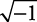has no real answer. The symbol i is created to representand is called an imaginary value. Since, i 2 = –1. Any expression that is a product of a real number with i is called a pure imaginary number.

##### Example 1

Simplify each of the following.

1.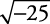2.3. (6 i)(4 i)

4.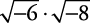1.2.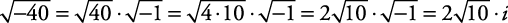3. This last expression is commonly written as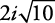so that the i is not mistakenly written under the radical.

4. (6 i)(4 i) = 24 i 2 = 24(–1) = –24

5.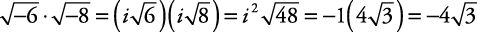For this last example, all imaginary values had to be put into their “ i‐form” before any simplifying could be done. Note that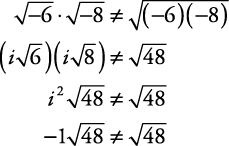That is, the product rule for radicals does not hold (in general) with imaginary numbers.

When i is raised to powers, it has a repeating pattern.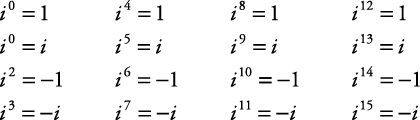When i is raised to any whole number power, the result is always 1, i, –1 or – i. If the exponent on i is divided by 4, the remainder indicates which of the four values is the result.

##### Example 2

Simplify each of the following.

1. i 34

2. i 95

3. i 108

4. i 53

1. i 34

Since 34 divided by 4 has a remainder of 2, i 34 = i 2 = –1. Think of it as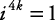, so2. i 95

Since 95 divided by 4 has a remainder of 3, i 95 = i 3 = – i.

3. i 108

Since 108 divided by 4 has a zero remainder, i 108 = i 0 = 1.

4. i 53

Since 53 divided by 4 has a remainder of 1, i 53 = i 1 = i.

Complex numbers and complex conjugates. A complex number is any expression that is a sum of a pure imaginary number and a real number. A complex number usually is expressed in a form called the a + bi form, or standard form, where a and b are real numbers. The expressions a + bi and abi are called complex conjugates. Complex conjugates are used to simplify the denominator when dividing with complex numbers. It is equivalent to rationalizing the denominator when dealing with fractions. The objective is to not end up with a complex number in the quotient's denominator.

Arithmetic with complex numbers is done in a similar manner as arithmetic with polynomials. The following are definitions for arithmetic with two complex numbers called ( a + bi) an d ( c + di).

Combining like terms and factoring out the i,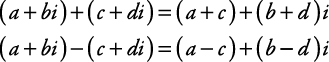Using the distributive property,Simplifying the denominator,##### Example 3

Find the sum, difference, product, and quotient of (4 + 3 i) and (5 – 4 i).Quotient: Rationalize the denominator.##### Example 4

Simplify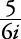.

Since 6 i is 0 + 6 i, its complex conjugate is 0 – 6 i. Therefore,Back to Top
A18ACD436D5A3997E3DA2573E3FD792A